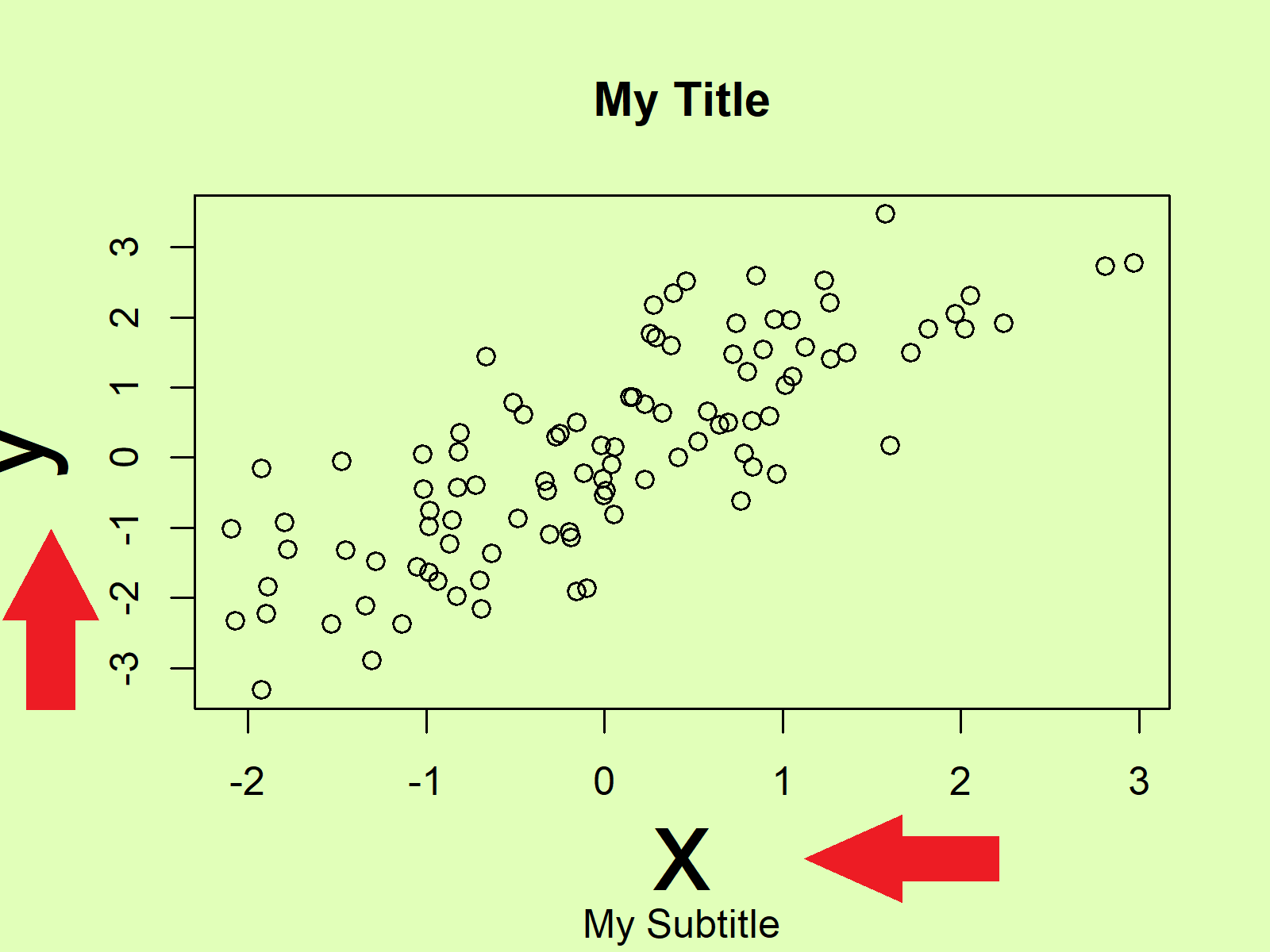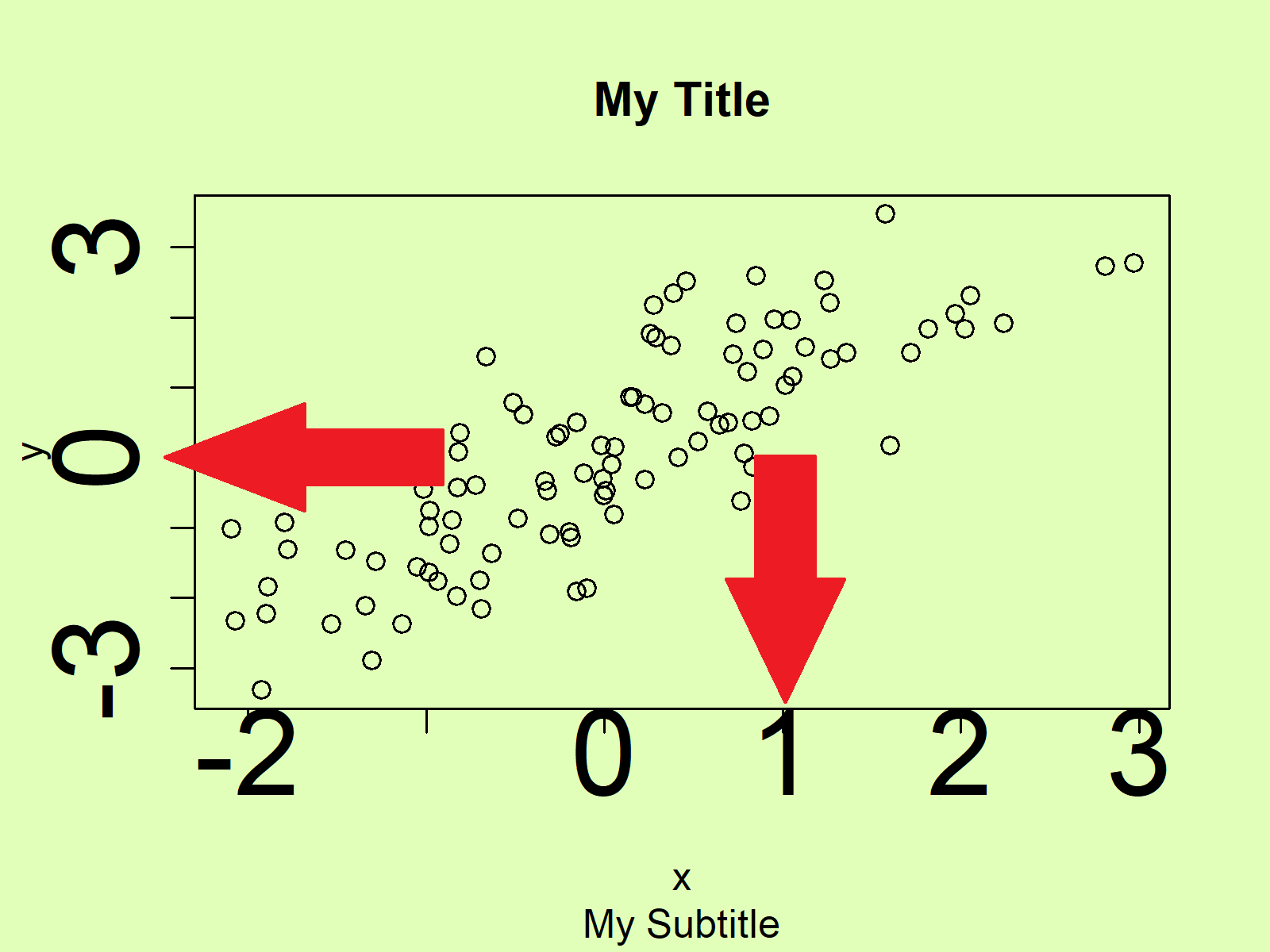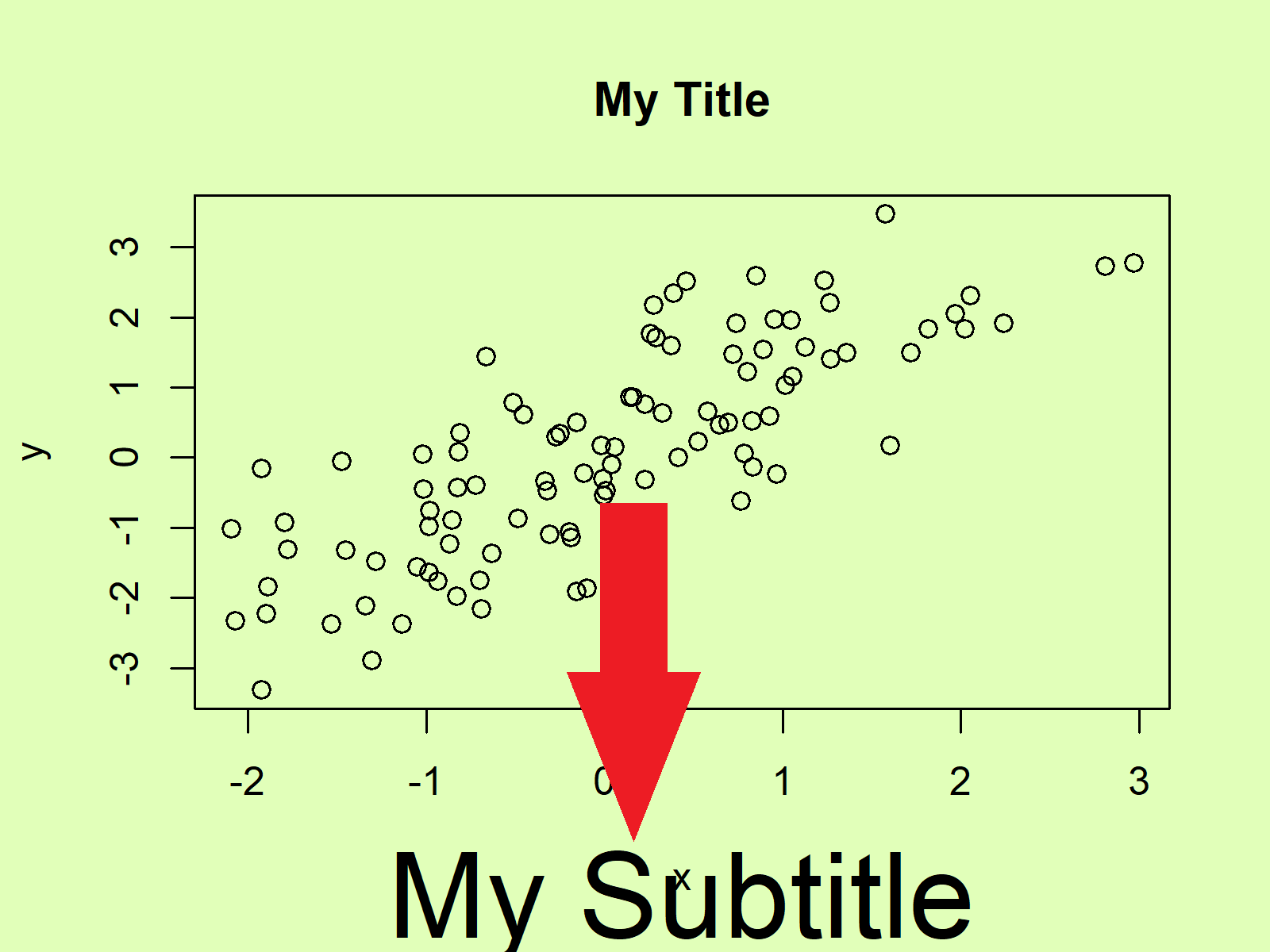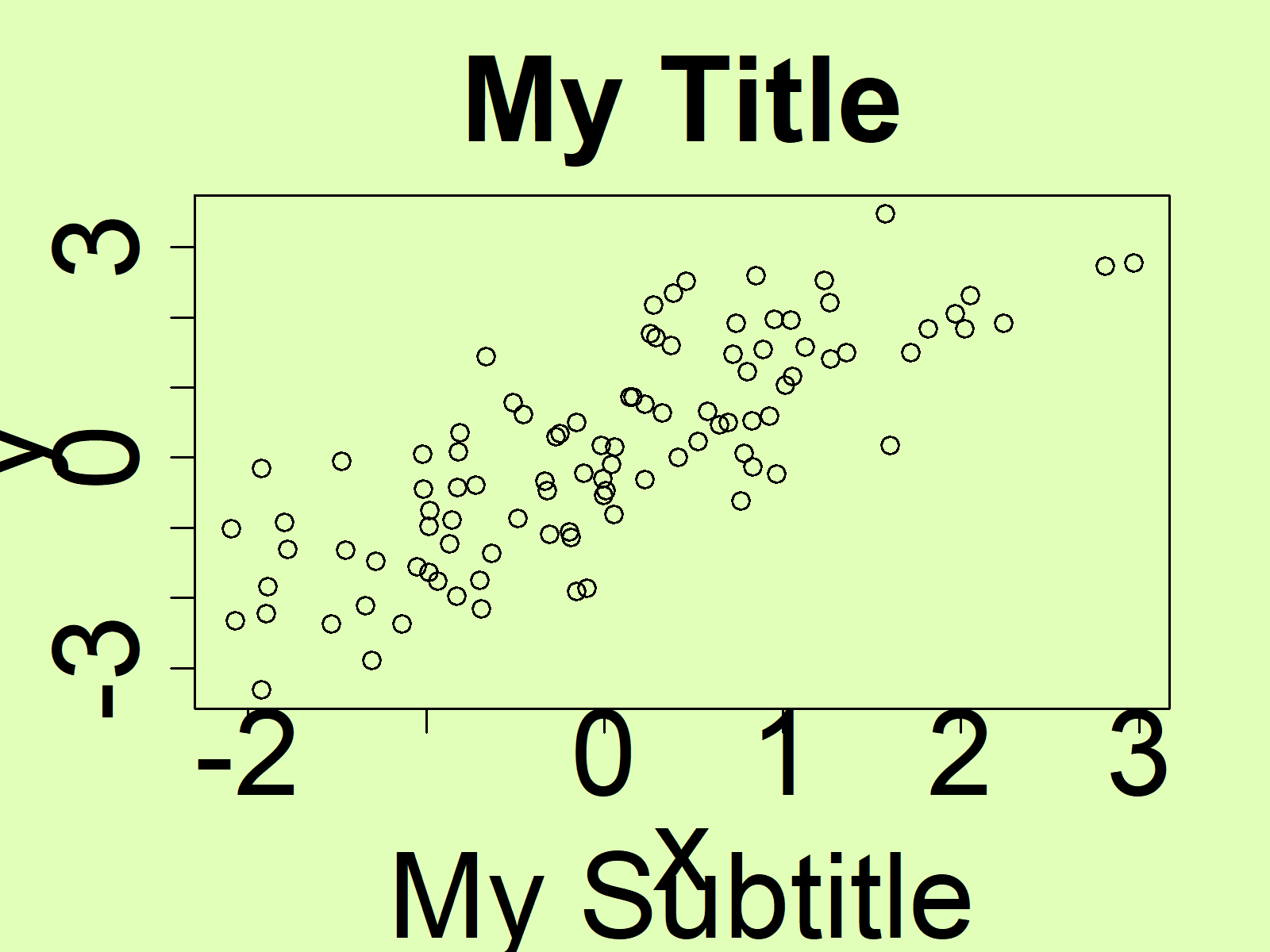# Increase Font Size in Base R Plot (5 Examples)

In this article you’ll learn how to increase font sizes in a plot in the R programming language.

The page contains these contents:

Here’s how to do it:

## Creation of Example Data

In the examples of this tutorial, I’ll use the following data:

```set.seed(7531)                                    # Create random data
x <- rnorm(100)
y <- x + rnorm(100)```

We can create a plot with default font sizes as follows:

```plot(x, y,                                        # Default plot
main = "My Title", sub = "My Subtitle")```Figure 1: Base R Plot with Default Font Sizes.

Now, if we want to increase certain font sizes, we can use the cex arguments of the plot function. Have a look at the following examples…

## Example 1: Increase Font Size of Labels

We can increase the labels of our plot axes with the cex.lab argument:

```plot(x, y,                                        # Increase label size
main = "My Title", sub = "My Subtitle",
cex.lab = 3)```Figure 2: Base R Plot with Increased Font Size of Labels.

## Example 2: Increase Font Size of Axes

The axis text can be increased with the cex.axis argument:

```plot(x, y,                                        # Increase axis size
main = "My Title", sub = "My Subtitle",
cex.axis = 3)```Figure 3: Base R Plot with Increased Font Size of Axes.

## Example 3: Increase Font Size of Main Title

The font size of the main title can be increased with the cex.main argument:

```plot(x, y,                                        # Increase title size
main = "My Title", sub = "My Subtitle",
cex.main = 3)```Figure 4: Base R Plot with Increased Font Size of Main Title.

## Example 4: Increase Font Size of Subtitle

The font size of the subtitle is getting larger by specifying a larger value for the cex.sub argument:

```plot(x, y,                                        # Increase subtitle size
main = "My Title", sub = "My Subtitle",
cex.sub = 3)```Figure 5: Base R Plot with Increased Font Size of Subtitle.

## Example 5: Increase Font Size of All Text

And finally, if we want to increase all font sizes of all plot elements, we can apply all cex arguments simultaneously:

```plot(x, y,                                        # Increase all text sizes
main = "My Title", sub = "My Subtitle",
cex.lab = 3,
cex.axis = 3,
cex.main = 3,
cex.sub = 3)```Figure 6: Base R Plot with Increased Font Size of All Text Elements.

Note that I always specified the cex arguments to be equal to 3. You may change this value to whatever value you want. The larger the cex value gets, the larger is the font size. You may also decrease the font size.

## Video & Further Resources

I have recently published a video on my YouTube channel, which shows the contents of this article. You can find the video below.

Please accept YouTube cookies to play this video. By accepting you will be accessing content from YouTube, a service provided by an external third party.If you accept this notice, your choice will be saved and the page will refresh.

Furthermore, I can recommend reading some of the other tutorials on my homepage:

Summary: This tutorial illustrated how to adjust text sizes in a plot in R programming.

In this tutorial, we have used a scatterplot to illustrate how to increase font sizes. Please note that we could apply the same kind of code to other types of graphics such as boxplot names, histograms, density plots, line charts, and so on.

Subscribe to the Statistics Globe Newsletter

•Vanessa
May 13, 2021 9:17 am

Hi, I was wondering what changes there would be to the code if I only wanted to change the font size of the X axis but not the Y axis. I’m plotting categorical data where variables report a range of income. For example: “\$150,000 or more.”

The result is that my X axis is very crowded, so I wanted to see if I could somehow isolate the text size of the x axis.

Thanks so much for your videos by the way. I’m a college student and I learned a lot of R code from you–so much that I have an A in the class (and I was initially afraid to take it). Anyway, thanks again.

•May 13, 2021 1:54 pm

Hi Vanessa,

First of all, thank you very much for this awesome feedback, and congratulations on your A-grade! 🙂

Regarding your question: You can change only the x-axis size using the axis() function. See the following example code:

```plot(x, y,                                        # Increase axis size
main = "My Title", sub = "My Subtitle",
xaxt = "n")
axis(1, cex.axis = 3)```

I hope that helps!

Joachim

•synat Keam
March 30, 2022 5:02 am

Hi Jo,

Thanks for putting these beautiful codes. I am wondering whether there are ways to combine base R plot and save base R plot for 300 dpi.

Kind Regards,

Synat

•March 30, 2022 6:57 am

Hey Synat,

Thank you, glad you like the tutorial!

There are many ways to export Base R plots to files. For instance, you may use the pdf() function to save your plot as a PDF. Within the pdf() function, you may use the width and height arguments to change the size of the output:

```pdf("my_plot.pdf", width = 20, height = 10)
plot(1:10)
dev.off()```

Regards,
Joachim

•Franco Kato
December 15, 2022 7:18 pm

Hi Joachim, thank you very much for the tutorials. I’m new to R and find them very helpful. My combination plot has no title. How do I modify/reduce the font size of the x-axis?

Note: I used the following code to come up with the plot: aggr(data, numbers=TRUE, prop=FALSE)

•Franco Kato
December 15, 2022 7:24 pm

Please mote that my x-axis is text

•December 19, 2022 10:06 am

Hello Franco,

Could you please tell me what kind of plot and data you are dealing with? Is it a scatter plot like in the tutorial? What is the text data that you use on the x-axis?

Regards,
Cansu

•Franco
December 20, 2022 7:08 am

Hi, It is a combinations plot. The data on the x-axis comprises brands of different beers. I managed to fix the problem when I re-run the code with a bigger window. Thx.

However I have another issue with the clustering algorithm. How can I fix messy dendrogram?

•December 20, 2022 9:14 am

Hello Franco,

It is usually an issue when you work with rather big datasets. Check the link. There different dendrogram configurations are provided.

Regards,
Cansu

•Nangula
April 11, 2023 6:19 pm

Thank you very much for the useful post. I am doing some random forest modelling with time series and because the names of my images which correspond to a month, and the dates are quite longer, and therefore are cutting. When I reduce the cex.axis, the labels are getting quite too small and unreadable. Is there a way to increase the margins of the entire plot or what can I do to make sure my labels fit very well and they don’t cut?

•Nangula
April 12, 2023 7:29 am

Hi Cansu,

I am using plot.

Here is my script:

plot(Importance_76_100_train__2019_FIX, xlab = “”, xaxt = “n”)
lz<-lapply(1:ncol(Importance_76_100_train__2019_FIX\$ImpHistory),function(i)
Importance_76_100_train__2019_FIX\$ImpHistory[is.finite(Importance_76_100_train__2019_FIX\$ImpHistory[,i]),i])
names(lz) <- colnames(Importance_76_100_train__2019_FIX\$ImpHistory)
Labels <- sort(sapply(lz,median))
axis(side = 1,las=2,labels = names(Labels),
at = 1:ncol(Importance_76_100_train__2019_FIX\$ImpHistory),cex.axis = 0.3)

•